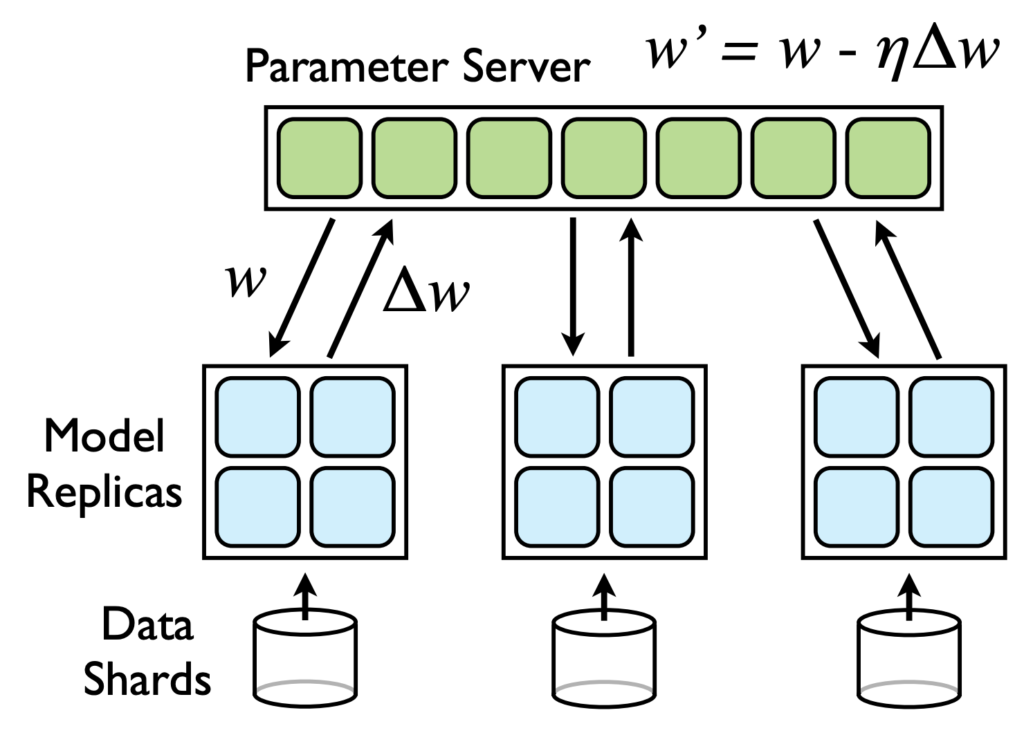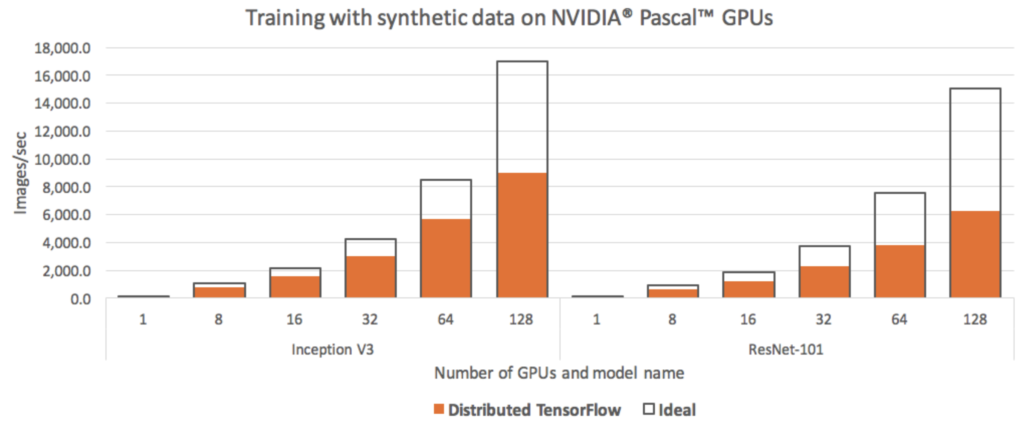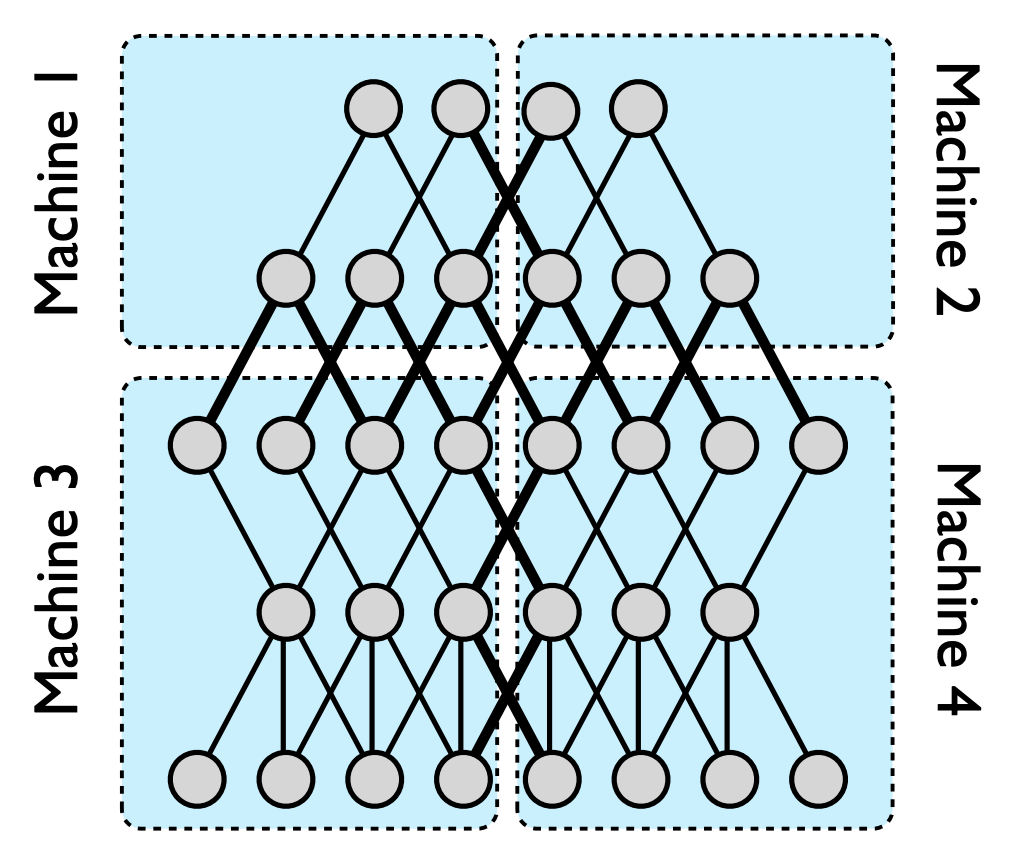# An introductory guide on distributed training of neural networks

## Introduction

The advent of complex deep learning models, which range from millions to billions of parameters, opened in recent years, the field of Distributed Deep Learning (DDL). DDL is primarily concerned with methods to improve the training and inference of deep learning models, especially neural networks, thru distributed computing.

Until the 1980s, neural networks were a niche topic, of limited interest only in academia. This is mostly because there wasn’t a practical interest in it. Training neural networks was very tedious, even for small architectures. This was due, in part, to the lack of a general theoretical framework behind NN’s training, but also the lack of algorithms that could use the inherent layer parallelism. Starting with Yann le Cun , who offered around this time the theoretical foundations behind backpropagation, neural networks have gradually gained in popularity. So much so that nowadays, hardware providers offer custom architectures for deploying neural networks, either on custom, high performance, GGPU boards or FPGA.

The main challenge of DDL is finding those models and procedures that mitigate mainly two problems:

• The inherent nature of machine learning algorithms to be iterative: because they represent optimization procedures (eg. finding the point of minimum error) the vast majority of algorithms repeat some sort of fixed-point update routines until convergence . For a single process the update rule might look similar to: $$\theta_{t+1} = \theta_t + \Delta (\mathcal{D}, \theta_t)$$ ($$\theta$$ being the model’s parameters, $$\Delta()$$ the update rule of the model and $$\mathcal{D}$$ the training data), while for multiple processes similar to: $$\theta_{t+1} = \theta_t + \sum_{p=1}^P \Delta (\theta_t, S_p(\theta_t))$$ (P being the number of parallel processes/workers and $$S_p$$ the purely sequential block).
• The communication overhead. As the number of concurrent workers is increased, more and more messages are passed between them (either to update their internal state with results computed by other workers, either stop from executing while they push the messages to a distributed messages queue). This behaviour is additive, to the point there is no gain in adding more workers.

Various techniques for distributing the load have been developed for the aforementioned problems. All are different forms of the two approaches to parallelizing the computation:

• Model parallelism: Different machines are responsible for computing a different part of the model (ex. assign each layer of a neural network to a different worker).
• Data parallelism: The same model is shared between the workers but the incoming data is different for each of them (ex. splitting the training data equally between the workers).
• It’s worth mentioning that here, by model, we don’t understand a fixed configuration of the model parameters(ex. all the weights in a neural network), but rather a model architecture (ex. the actual structure of the neural network).

This report presents a summary of DDL methods, starting with the foundational work of Jeffrey Dean et al, in Large Scale Distributed Deep Networks , what drawbacks those methods had, how they were solved, along with other significant papers, that presented new, model-centric approaches in DDL , .

## The iterative nature of ML algorithms

### The backpropagation algorithm

To properly understand the limits in training distributed neural networks a solid background behind the algorithm of backpropagation(BP) is needed. The algorithm solves the problem of transmitting an error inside the neural network, starting from the last layer. For this algorithm to work, the activation functions must be differentiable.

Although this algorithm might seem exponential with the number of layers in reality is only quadratic. The reason is at any step of the algorithm we look, locally, only at two layers (the layer that must be updated and the previous layer).

Mathematically, the scope of the backpropagation is computing the term $$(\frac{\partial E}{\partial w_{ij}})^l$$, $$E$$ being the expected error computed at the last layer and $$\boldsymbol l$$ being the index of the layer in which the weight $$\boldsymbol i$$ of neuron $$\boldsymbol j$$ is.

Below are the two equations of the backpropagation algorithm. $$e$$ is the error between the predicted and actual target, $$o$$ is the output of the activation function and $$\Sigma$$ the output of the summation part, before applying the activation.

$$\frac{\partial e}{w_{ij}} = \frac{\partial e}{\partial o_j}\dfrac{\partial o_j}{\partial \Sigma_j}\frac{\partial \Sigma_j}{w_{ij}}$$

$$\frac{\partial e}{\partial o_j} = \sum_{l\in L+}\frac{\partial e}{\partial o_l}\frac{\partial o_l}{\partial \Sigma_l}\frac{\partial \Sigma_l}{o_j}$$

### Improving backpropagation with delayed gradients

The backpropagation algorithm, although it revolutionized the training of neural networks, doesn’t come without some disadvantages:

• In it’s basic form, when used with stochastic gradient descent(SGD), the model converges slowly and it’s prone to getting stuck in local minimas and saddle regions.
• It is difficult to improve the training time, as you can’t pipeline the training instances right out of the box. For example, suppose we have two training vectors corresponding to class 1 and 0 fed into the network one after the other in a pipelined fashion. When the first one reaches the output, the backpropagation will start, going backwards to update the weights. But the weights will be updated according to the effect the other class produced.

In the above image each module is a stack of layers. Backpropagation algorithm running the forward pass (from 1 to 3) and backward pass (from 4 to 6) in sequential order. For example, module A cannot perform step 6 before receiving $$\delta^t_A$$ which is an output of step 5 in module B .

Zhouyuan Huo et al.  present a simple architecture that can circumvent this problem and speedup the bacpropagation phase. Their idea is to split a neural network into $K$ blocks with $K-1$ additional states to hold the gradients from the previous K-1 iterations, depending on the block (block closer to output will have states closer to present and vice-versa). Because of this, they’ve coined this method Delayed Gradient Update and although it’s not as precise as the original backpropagation algorithm, it offers a speedup of K in the backpropagation phase.

## Data parallelism

### Parameter averaging

Parameter averaging is conceptually, the simplest approach to data parallelism. The core idea is to split the training data between the available workers, which hold the same model, and train them independently. After they have trained for a controlled amount of time, all the models are collected and the weights are averaged between the workers (i.e. if we have K workers, the $$j$$-th weight from the $$l$$-th layer will be $$\frac{1}{K}\sum_{k=1}^K w^{(l)}_{jk}$$). More precisely, the update rule will look as follows:

$$W_{i+1} = \frac{1}{K}\sum_{k=1}^{K}W_{i+1,k}\ = \frac{1}{K}\sum_{k=1}^{K}\left( W_i – \frac{\alpha}{m}\sum_{j=(k-1)m+1}^{km}\frac{\partial e^j}{\partial W_i}\right)\ = W_i – \frac{\alpha}{nm}\sum_{j=1}^{nm}\frac{\partial e^{j}}{\partial W_i}$$

The equation above shows that parameter averaging is actually equivalent with a single worker that receives more data.

Parameter averaging is an example of a synchronous distributed SGD because at the end of the update phase, all the workers receive an updated copy of the weights. From a distributed systems point of view this is an example of MapReduce , the mapping being applied by each worker resulting in a new model and the reduce being the average operation applied over the resulting models by the parameter server.

Although parameter averaging seems like a promising idea, in reality it suffers from two communication bottlenecks:

• Because it’s necessary to average the models coming from the workers this will imply it will be as fast as the slowest worker.
• There isn’t a clear answer about the number of synchronizations the workers should make. Too many, and the workers would be constantly waiting for the averaged parameters. Too few, and the local parameters in each worker would diverge too much, resulting in a poor model after averaging. The intuition here is that the average of N different local minima are not guaranteed to be the global local minima.

Because of the problems mentioned above, the classic approach to parameter averaging is to use an averaging period (in terms of number of minibatches per worker) greater than 1.

This method is similar with parameter averaging, with the difference that instead of sending all the parameters to the parameter server, only the updates are sent and are applied directly to a copy of the model that is held by the parameter server as well. This is the fastest approach in doing parallel training of neural networks. The workers are free to decide when to update, but a consistent rule must be followed to minimize the effects of the stale gradient problem.

The stale gradients appear because the workers are not synchronized in any way. This means there is a chance for a worker to send a set of updates that have as baseline an “old” model from the parameter server. It doesn’t matter how many new updates have arrived at the parameter server, if the worker that sent the parameters hasn’t updated beforehand. It’s action will cancel all the updates the other workers made to the parameter server from the moment he took that baseline.

A naive implementation of asynchronous SGD can result is very high staleness values for the gradients. For example, Gupta et al.  showed that the average gradient staleness is equal to the number of executors. For N executors, this means that the gradients will be on average N steps out of date by the time they are applied to the global parameter vector. This has real-world consequences: high gradient staleness can slow network convergence significantly, and even stop some configurations from converging at all. Earlier async SGD implementations (such as Google’s DistBelief system ) did not account for this effect, and hence learning was considerably less efficient than it otherwise could have been.

### Downpour SGD

This is a variant of asynchronous stochastic gradient descent introduced in , the same paper that presented DistBelief. The basic approach is to divide the training data into a number of subsets and run a copy of the model on each of these subsets (i.e. data parallelism). The workers communicate updates through a centralized parameter server, which keeps the current state of all the parameters of the model, sharded across many machines.

This approach is asynchronous in two distinct aspects: the models replicas run independently of each other and the parameter server shards also run independently of one another.

Due to it’s asynchronous nature, Downpour SGD is more robust to machine failures than standard SGD. If one model replica fails, the system as a whole is not so affected as the new state can be recovered from one of remaining states.Downpour SGD: Model replicas asynchronously fetch parameters $$w$$ and push gradients $$\Delta w$$ to the parameter server

### Ring AllReduce paradigm. Horovod use-case.

Both data parallelization methods presented above (Parameter averaging and Asynchronous SGD) suffer from the same communication bottleneck. Because there is a single parameter server that behaves like a master process, all the other workers must update a common state. If the number of workers becomes very large this will essentially saturate the communication channel with sync and update messages.

The solution is using a ring topology, eliminating the parameter server altogether. This method is part of now a mature library, called Horovod , which leverages the communication between the GPUs, almost doubling the training speed in distributed environments(the effect being more noticeable in environments with a large number of CPUs).

Tbe Horovod library was inspired by a Baidu article  from early 2017 that promoted a different algorithm for averaging gradients and communicating those gradients to all nodes called Ring AllReduce. Their proposed algorithm was inspired from another paper published in 2009 by Patarasuk and Yuan .Multi-GPU scaling performance using TensorFlow. When comparing images processed per second while running the standard TensorFlow benchmarking suite on NVIDIA Pascal GPUs (ranging from 1 to 128) with both the Inception V3 and ResNet-101 TensorFlow models to theoretically ideal scaling (computed by multiplying the single-GPU rate by the number of GPUs), the hardware resources are not fully utilized  .

By properly scaling the GPU architecture in the proposed ring topology, it has been showed that an increase independent on the number of GPUs can be reached. For example, computing the column-wise sum of $$N$$ vectors, that have in total K elements, was shown to approach $$2(N-1)\frac{K}{N}$$.

A list with examples with the most popular frameworks that use Horovod can be found on https://github.com/uber/horovod/tree/master/examples.

## Model parallelism

By model parallelism we understand those situations where it’s possible to partition the network between different independent entities. This can be done either locally, by distributing the workload to different processes and threads, or if the model is very large and imposes it, to different machines in a distributed fashion.

Many model parallelism techniques are deeply linked with the topology of the neural network. For example, in networks that have fully connected layers, distributing the load between different machines adds a large communication overhead. More precisely, every neuron that is handled by a certain worker will have to pass messages to the worker handling the next layer.

The backpropagation phase is also inherently iterative, as described in chapter 2, thus making this process harder to speed up, especially when used with stochastic gradient descent.

### Intra and inter layer techniques. TensorFlow use-case.

One of the simplest improvements that can be made in order to speed up the training phase of a neural network comes from the observation that, in a given layer, the neurons are independent. This implies we can assign a different execution unit for each neuron for the computation of the sum and output. This translates to parallelising the matrix multiplications at the level of a layer.

A more complex scenario is when the network topology has some local structure between the layers. This implies there are neurons which are not connected in any way with other neurons, so higher level parallelism can be exploited. This feature has been first described and implemented in the DistBelief framework (TensorFlow’s precursor) .An example of model parallelism in DistBelief. A five layer deep neural network with local connectivity is shown here, partitioned across four machines (blue rectangles). Only those nodes with edges that cross partition boundaries (thick lines) will need to have their state transmitted between machines. Even in cases where a node has multiple edges crossing a partition boundary, its state is only sent to the machine on the other side of that boundary once. Within each partition, computation for individual nodes will the parallelized across all available CPU cores.

For example, in Tensorflow, all the operations that can be parallelized internally, such as matrix multiplication (tf.matmul()) or a reduction (e.g. tf.reduce_sum()). TensorFlow will execute them by scheduling tasks in a thread pool with intra_op_parallelism_threads threads. This configuration option therefore controls the maximum parallel speedup for a single operation. When running multiple operations in parallel, these operations will share this thread pool.

For operations that are independent in the TensorFlow graph—because there is no directed path between them in the dataflow graph, TensorFlow will attempt to run them concurrently, using a thread pool with inter_op_parallelism_threads threads. If those operations have a multithreaded implementation, they will (in most cases) share the same thread pool for intra-op parallelism .

The two configurations above are set using the tf.ConfigProto and passed to tf.Session in the config attribute as shown in the snippet below:

config = tf.ConfigProto()
tf.Session(config=config)

For both configuration options, if they are unset or set to 0, will default to the number of logical CPU cores .

## Conclusion

Distributed neural networks are not the swiss knife of neural networks when itcomes to training, their performance being deeply dependent on the nature of theproblem, the topology of the network and most importantly the model’s complexity.Nonetheless, if used judiciously, they can offer a massive increase in performance,reducing with more than an order of magnitude the training time of complex neuralnetworks that otherwise would require weeks to train.

## References

1. Kim et al., STRADS: A Distributed Framework for Scheduled Model Parallel Machine Learning
2. Yann Le Cun, A Theoretical Framework for Back-Propagation
3. Dean et al., Large Scale Distributed Deep Networks
4. Gupta, Suyog and Zhang, Wei and Wang, Fei, Model Accuracy and Runtime Tradeoff in Distributed Deep Learning: A Systematic Study
5. Rohan Anil et al, Large scale distributed neural network training through online distillation
6. Zhouyuan Huo, Bin Gu, Qian Yang, Heng Huang, Decoupled Parallel Backpropagation with Convergence Guarantee
7. Skymind Company, Distributed Deep Learning, Part 1: An Introduction to Distributed Training of Neural Networks